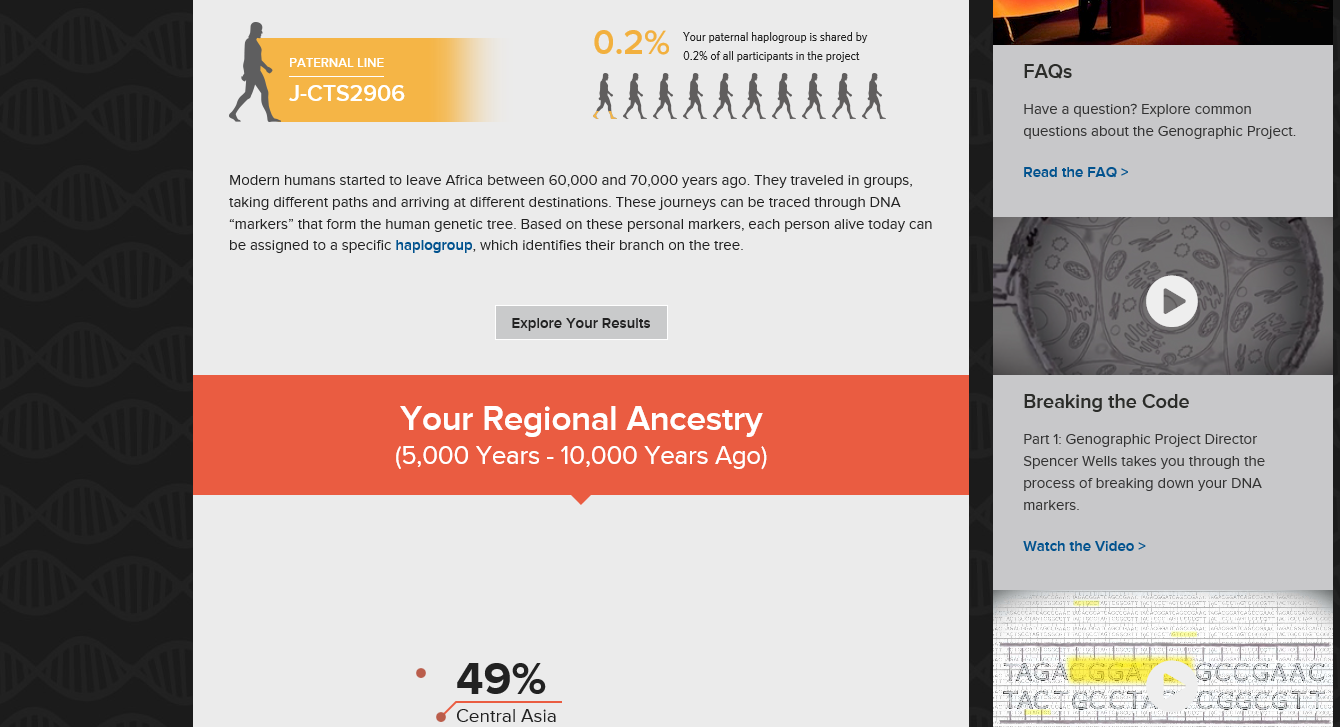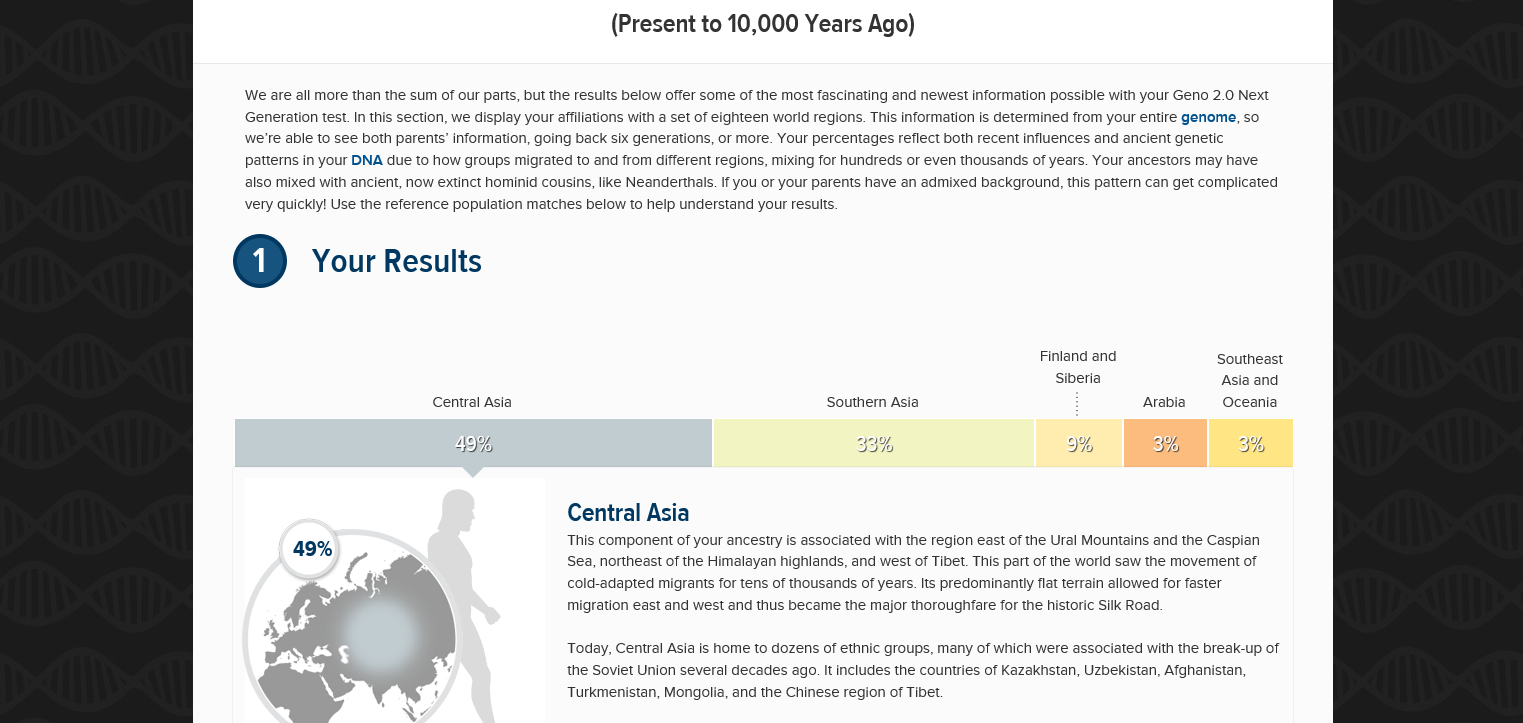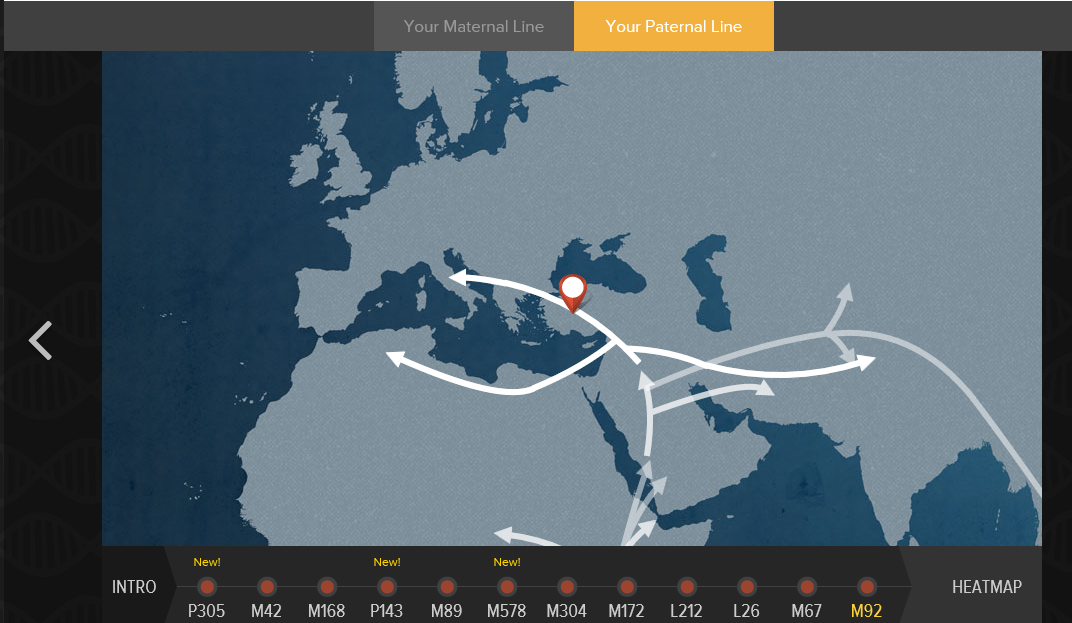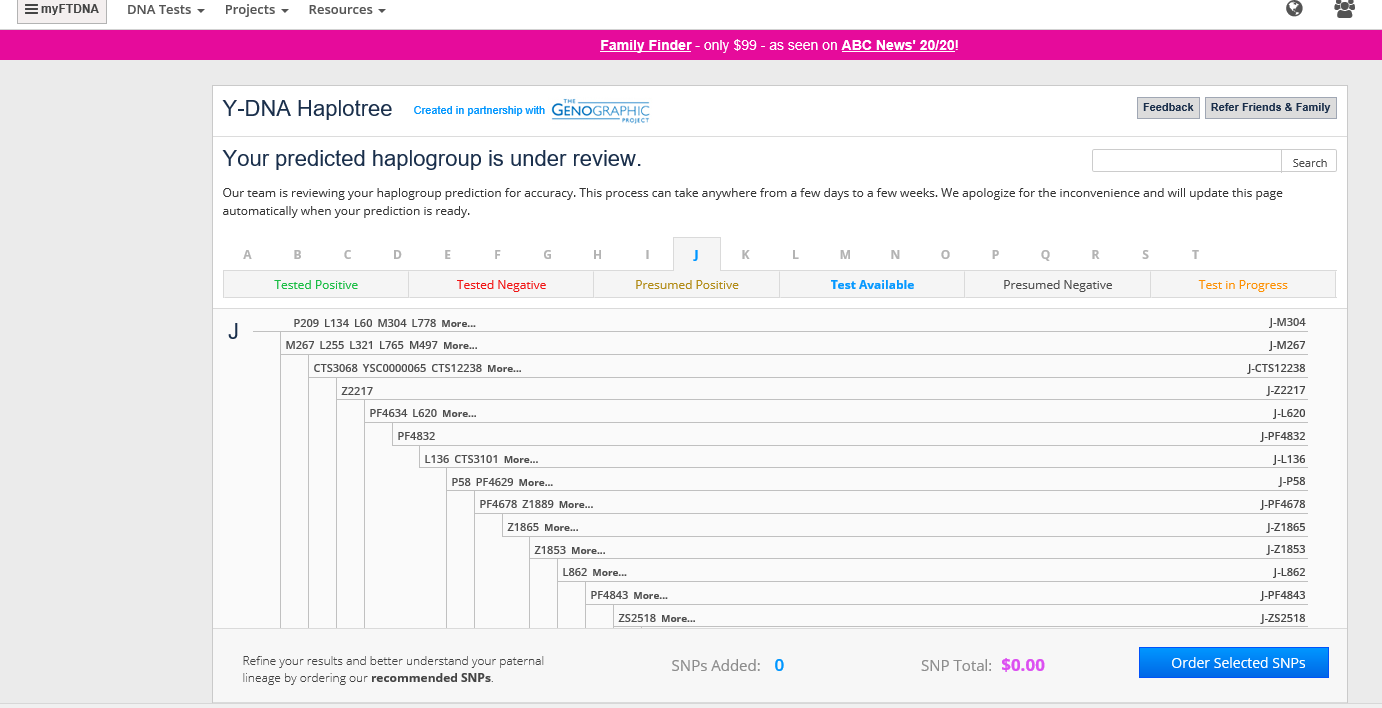Thread: Haplogroup J - CTS2906 Punjabi Jatt

1. Haplogroup J - CTS2906 Punjabi Jatt

Hi Folks ,

I would be requesting the experts for some input. National Geographic Geno 2.0 tested my DNA samples and identified by paternal haplogroup as J-CTS2906. Is this the same as J2-M92 or is it J2a1b1?

2d82v0p.jpg
2a9vdky.jpg

I would appreciate any guidance on this matter available. I have the raw snp file in CSV format. Is there anything I can do with it for extra information on my Haplogroup Type?

Thanks and regards,
Jatt2016Reply With Quote

2. The Following 3 Users Say Thank You to jatt2016 For This Useful Post:

Agamemnon (02-14-2016),  redifflal (02-14-2016),  rms2 (02-14-2016)

3. Other screen shots from my DNA report :
Capture.jpg

Capt1ure.jpgReply With Quote

4. The Following 2 Users Say Thank You to jatt2016 For This Useful Post:

Agamemnon (02-14-2016),  redifflal (02-14-2016)

5. Your SNP is a downstream of M92. You can see here:
http://www.yfull.com/tree/J-Z8096/
If you hover over the "+15 snps" box you can see that CTS2906 is the same as Z8096.Reply With Quote

6. The Following 3 Users Say Thank You to Viktor Reznov For This Useful Post:

Agamemnon (02-14-2016),  Awale (02-16-2016),  rms2 (02-14-2016)

7.Originally Posted by Viktor ReznovYour SNP is a downstream of M92. You can see here:
http://www.yfull.com/tree/J-Z8096/
If you hover over the "+15 snps" box you can see that CTS2906 is the same as Z8096.

Thanks a lot Victor and this is my raw data from family tree site. A question : why my DNA result show M92 then?

A531+, A91+, BY1142+, BY1357+, BY136_FGC19909_Z18229+, BY1551+, BY1585+, BY1734+, BY20+, BY2510+, BY65_Z18181+, BY763+, BY876+, CTS10031+, CTS10147+, CTS10188+, CTS10229+, CTS103+, CTS10300+, CTS10428+, CTS10433+, CTS10448+, CTS10552+, CTS10572+, CTS10615+, CTS10648+, CTS1066+, CTS10723+, CTS10745+, CTS10761+, CTS10821+, CTS10847+, CTS11012+, CTS11041+, CTS11071+, CTS11088+, CTS11126+, CTS11148+, CTS11190+, CTS11261+, CTS11354+, CTS114+, CTS1141+, CTS11436+, CTS11441+, CTS1148+, CTS11503+, CTS11530+, CTS1164+, CTS11651+, CTS1171+, CTS11710+, CTS11731+, CTS11759+, CTS11816+, CTS11907+, CTS11949+, CTS11970+, CTS12023+, CTS12058+, CTS1216+, CTS12449+, CTS12450+, CTS12578+, CTS1276+, CTS12856+, CTS12933+, CTS12934+, CTS12948+, CTS12950+, CTS12976+, CTS1340+, CTS1353+, CTS1413+, CTS1436+, CTS1633+, CTS1710+, CTS1758+, CTS1806+, CTS191+, CTS202+, CTS2067+, CTS210+, CTS2105+, CTS2134+, CTS2230+, CTS2275+, CTS2284+, CTS2289+, CTS2413+, CTS2488+, CTS2515+, CTS2670+, CTS278+, CTS2800+, CTS2848+, CTS2906+, CTS2947+, CTS305+, CTS316+, CTS3202+, CTS3268+, CTS3366+, CTS3403+, CTS3466+, CTS347+, CTS3519+, CTS352+, CTS3543+, CTS3654+, CTS3663+, CTS3700+, CTS3802+, CTS3837+, CTS3868+, CTS3902+, CTS4053+, CTS4086+, CTS4089+, CTS4094+, CTS4137+, CTS4178+, CTS4209+, CTS4235+, CTS4326+, CTS4406+, CTS4463+, CTS4608+, CTS4643+, CTS4715+, CTS4781+, CTS4784+, CTS4793+, CTS4853+, CTS4984+, CTS5052+, CTS5152+, CTS5156+, CTS5254+, CTS5304+, CTS5334+, CTS5359+, CTS5370+, CTS5649+, CTS5690+, CTS58+, CTS5828+, CTS585+, CTS5860+, CTS5933+, CTS5939+, CTS597+, CTS5998+, CTS6062+, CTS6063+, CTS6090+, CTS6117+, CTS6265+, CTS6285+, CTS6352+, CTS636+, CTS6365+, CTS6394+, CTS6403+, CTS6438+, CTS6447+, CTS6449+, CTS6468+, CTS6481+, CTS6506+, CTS656+, CTS6613+, CTS6631+, CTS6677+, CTS6704+, CTS6848+, CTS687+, CTS6918+, CTS6967+, CTS7022+, CTS7101+, CTS7147+, CTS7186+, CTS7193+, CTS7227+, CTS7266+, CTS7275+, CTS7285+, CTS7299+, CTS730+, CTS7317+, CTS7335+, CTS736+, CTS7451+, CTS7598+, CTS7601+, CTS7611+, CTS7626+, CTS7642+, CTS7767+, CTS7803+, CTS7810+, CTS7925+, CTS7929+, CTS7942+, CTS8027+, CTS8126+, CTS8203+, CTS8216+, CTS8300+, CTS8440+, CTS8492+, CTS8506+, CTS8521+, CTS860+, CTS8645+, CTS8723+, CTS8815+, CTS9056+, CTS9154+, CTS9240+, CTS9322+, CTS9325+, CTS9335+, CTS9387+, CTS9471+, CTS9518+, CTS9525+, CTS9539+, CTS9558+, CTS97+, CTS9716+, CTS9894+, CTS9900+, CTS9925+, CTS9975+, CTS9976+, DF109_FGC4101_S660_Y2845+, F1007+, F1013+, F1024+, F1055+, F1096+, F1108+, F1127+, F1159+, F1207+, F1221+, F1291+, F1302+, F1367+, F1405+, F1412+, F142+, F1442+, F1474+, F1476+, F1539+, F1540+, F1567+, F1594+, F1703+, F1712+, F1796+, F1813+, F1848+, F1874+, F188+, F1899+, F1924+, F1946+, F1956+, F2018+, F202+, F2028+, F2132+, F2149+, F2165+, F2208+, F2224+, F2236+, F2304+, F2332+, F2343+, F2370+, F2371+, F240+, F2480+, F2516+, F2546+, F2558+, F2600+, F2654+, F2724+, F2742+, F2753+, F2761+, F2767+, F2840+, F2850+, F287+, F2873+, F2924+, F2947+, F2960+, F2961+, F299+, F2990+, F3048+, F3056+, F3057+, F3108+, F3143+, F3195_PF1806+, F3270+, F3368_PF3566+, F3402_PF3567+, F3422+, F3444+, F3451+, F3491+, F3518+, F3582+, F3595+, F3625+, F3632+, F3692+, F3697+, F371+, F3735+, F3739+, F3806+, F386+, F3869+, F3892+, F3907+, F3949+, F3956+, F3994+, F4003+, F4042+, F4111+, F4176+, F4188_PF3539+, F4244+, F4251+, F4257+, F4282+, F4338+, F489+, F492+, F526+, F557+, F565+, F61+, F646+, F716+, F719+, F736+, F741+, F743+, F819+, F836+, F837+, F862+, F896+, F910+, F995+, FGC11678+, FGC11897_YP327+, FGC12120+, FGC12627_Y3429+, FGC13364_Y3138+, FGC16362_Z18162+, FGC16683_Y5619+, FGC1721_Y5322+, FGC20866_ZS2566+, FGC20874_ZS2572+, FGC24646+, FGC28639_YP556+, FGC29572+, FGC3718_Y6369+, FGC6948_Y2703+, K257+, K386+, L104+, L1093+, L1186+, L1247+, L132+, L1324+, L167+, L183+, L187+, L213+, L245+, L253+, L254+, L294+, L325+, L387+, L440+, L442+, L453+, L459+, L464_1+, L484+, L492+, L496+, L498+, L503+, L533+, L542+, L551+, L552+, L556+, L557+, L625+, L636+, L665+, L72+, L782+, L784+, L817+, L840+, L99+, M102+, M116+, M129+, M139+, M171+, M251+, M262+, M275+, M288+, M367+, M39+, M69+, M89+, M94+, M9574+, M9588+, MC14+, N4+, P102+, P108+, P117+, P118+, P129+, P135+, P136+, P141+, P145+, P148+, P151+, P159+, P160+, P166+, P181+, P194+, P202+, P268+, P269+, P289+, P305+, P40+, P52+, P59+, P77+, P80+, P84+, P91+, PAGES00010+, PAGES00048+, PAGES00049+, PAGES00081+, PAGES00101+, PAGES00105+, PF1015+, PF1026+, PF1031+, PF1085+, PF1097+, PF1141+, PF1147+, PF1152+, PF1164+, PF1169+, PF121+, PF1226+, PF1232+, PF1252+, PF1269+, PF1270+, PF1279+, PF1283+, PF133+, PF1368+, PF147+, PF156+, PF1562+, PF1577+, PF1587+, PF22+, PF228+, PF2334+, PF2436+, PF2438+, PF2466+, PF2481+, PF2495+, PF2579+, PF2593+, PF2611+, PF2624+, PF2635+, PF2643+, PF276+, PF288+, PF293+, PF302+, PF3051+, PF3086+, PF3107+, PF3188+, PF3220+, PF3248+, PF328+, PF3298+, PF331+, PF3320+, PF3561+, PF3823+, PF3890+, PF3964+, PF3986+, PF40+, PF4105+, PF4193+, PF4246+, PF4533+, PF4573+, PF4576+, PF4589+, PF4592+, PF4647+, PF4653+, PF4720+, PF4876+, PF4888+, PF4957+, PF5014+, PF5104+, PF5122+, PF5123+, PF5125+, PF5147+, PF535+, PF5381+, PF5436+, PF5517+, PF5735+, PF5744+, PF5784+, PF5787+, PF5792+, PF5815+, PF5819+, PF601+, PF6063+, PF625+, PF6275+, PF6286+, PF6300+, PF6314+, PF6369+, PF644+, PF6673+, PF668+, PF671+, PF679+, PF6864+, PF6865+, PF6914+, PF698+, PF700+, PF7067+, PF7328+, PF7341+, PF7374+, PF7434+, PF747+, PF7527+, PF7552+, PF7557+, PF791+, PF794+, PF806+, PF829+, PF888+, PF907+, PF926+, PF93+, PF946+, PF95+, PF966+, PF968+, PF971+, PF997+, PK1+, PR805+, rs12116413+, rs16980396+, rs16980499+, rs17842387+, rs2032652+, rs2075640+, rs35249440+, rs35407486+, rs7067237+, rs7892924+, rs7893044+, rs9306845+, rs9306848+, rs9785670+, rs9785743+, rs9785853+, rs9785905+, rs9786082+, rs9786181+, rs9786247+, rs9786290+, rs9786325+, rs9786774+, S3207+, s5153+, S7123+, SK1247_Y4915+, SK1410_Y5528_Z20169+, V174+, V187+, V21+, V216+, V221+, V227+, V250+, V29+, V32+, V59+, V78+, V79+, V8+, V90+, V94+, Y1038+, Y1049+, Y1083+, Y1113+, Y1122+, Y32+, Y4864_Z16422+, Y5272+, Y5305_Z16500+, Y5759_Z16420+, Y6181+, Y763+, YP321+, YSC0000081+, YSC0000150+, YSC0000216+, YSC0000292+, YSC0001071+, Z11180+, Z1149+, Z130+, Z133+, Z138+, Z14303+, Z1456+, Z1476+, Z148+, Z1483+, Z1504+, Z1518+, Z1589+, Z1593+, Z1616+, Z18133+, Z18140+, Z1817+, Z18186+, Z1835+, Z187+, Z189+, Z1975+, Z2069+, Z2082+, Z2094+, Z213+, Z2396+, Z269+, Z270+, Z274+, Z2970+, Z2976+, Z2983+, Z30+, Z315+, Z319+, Z347+, Z350+, Z3723+, Z39+, Z477+, Z515+, Z531+, Z544+, Z60+, Z625+, Z638+, Z72+, Z75+, Z767+, Z966+, ZS1727+, ZS251+Reply With Quote

8. The Following User Says Thank You to jatt2016 For This Useful Post:

Agamemnon (02-14-2016)

9.Originally Posted by Viktor ReznovYour SNP is a downstream of M92. You can see here:
http://www.yfull.com/tree/J-Z8096/
If you hover over the "+15 snps" box you can see that CTS2906 is the same as Z8096.
Hi Viktor :

Just noticed on your profile info that you have M92 too? Does this mean that we share the same paternal DNA. Pardon my ignorance, I am new to this DNA thing and my question might be a dumb one. I am trying to understand things here.

Thanks and regards,

Jatt2016Reply With Quote

10. Briefly looking at your results, I believe you are somewhere around H-M69. FTDNA has problems. You need to contact FTDNA and Geno 2.0 and cc: [email protected]. You are not in the J haplogroup!Reply With Quote

11.Originally Posted by Ali16Briefly looking at your results, I believe you are somewhere around H-M69. FTDNA has problems. You need to contact FTDNA and Geno 2.0 and cc: [email protected]. You are not in the J haplogroup!
The Haplogroup that was provided to me is by Nat Geo and not FTDNA....check screen shots below :Reply With Quote

12. Is that a full list of positive SNPs ? As Ali mentioned there might be some problem in haplogroup assignment . You doesn't seem to be positive for M172 and M410 which are defining SNPs for J2 and J2a respectively but you are positive for M69 which is defining SNP for haplogroup HReply With Quote

13. As far as I can tell, your subclade under CTS2906 is J2a-L556. It's a major jewish subclade of M92 IIRC.Reply With Quote

14. The Following User Says Thank You to Agamemnon For This Useful Post:

Awale (02-16-2016)

15.Originally Posted by BMGIs that a full list of positive SNPs ? As Ali mentioned there might be some problem in haplogroup assignment . You doesn't seem to be positive for M172 and M410 which are defining SNPs for J2 and J2a respectively but you are positive for M69 which is defining SNP for haplogroup H
National Geographic has classified me as J-CTS2906 and if u see the red dots above you would see M172 and M92 there. I have transferred my results to FTDNA and they are reviewing the raw file to classify me. The tested positives should be green, am I right?Reply With QuotePosting Permissions

• You may not post new threads
• You may not post replies
• You may not post attachments
• You may not edit your posts
•